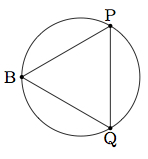# Inscribing an equilateral triangle in a circle

Alignments to Content Standards: G-CO.D.13

Suppose we are given a circle of radius $r$. The goal of this task is to construct an equilateral triangle whose three vertices lie on the circle. Suppose $\overline{AB}$ is a diameter of the circle. Draw a circle with center $A$ and radius $r$ and label the two points of intersection of the circles $P$ and $Q$ as pictured below:1. Show that $m(\angle ABP) = 30$ and $m(\angle ABQ) = 30$.
2. Show that $\triangle PBQ$ is an equilateral triangle inscribed in the given circle.

## IM Commentary

Constructing an equilateral triangle inscribed in a circle requires creativity and a good knowledge of geometry and/or trigonometry. The approach taken in the solution here uses the fact that $\sin{30} = \frac{1}{2}$. Hence in order to construct a 30 degree angle it is sufficient to be able to construct a right triangle whose hypotenuse has twice the length of a leg. Students will need to know that $\angle APB$ is right since it is inscribed in a circle with one ray along a diameter of the circle. The ratio of $(2:1)$ for the hypotenuse to the leg is arranged by using the diameter of a circle for the hypotenuse and the radius of a congruent circle for the leg.

This task implements many important ideas from geometry including trigonometric ratios, important facts about triangles, and reflections if students choose to view $\triangle ABQ$ as the reflection of $\triangle ABP$ about $\overleftrightarrow{AB}$. As a result, it is recommended that this task be undertaken relatively late in the geometry curriculum. It could also be given in open ended form , that is requesting to produce an inscribed equilateral triangle within a given circle without suggesting an approach or providing a picture. An alternative method begins by noting that if $O$ is the center of the original circle, then $\triangle AOP$ is equilateral (all three sides are radii of congruent circles). We can continue drawing circles, with centers $P$ and $Q$ and each time we get another equilateral triangle. The end result will be a regular hexagon inscribed inside the circle: joining every other vertex of this hexagon gives an equilateral triangle inscribed in the circle. A variant of this argument, focused only on the hexagon, is presented in http://www.illustrativemathematics.org/illustrations/786.

Work on this task can develop skills related to MP1, Make Sense of Problems and Persevere in Solving Them, and also MP3, Construct Viable Arguments and Critique the Reasoning of Others. In the first case, the argument is complex and requires putting together several abstract arguments which need to be related to the construction of the desired equilateral triangle. Because of this complexity, it is ideally suited for group work so that students can combine their strengths and skills.

## Solution

1. We know that $|AP| = r$ because it is a radius of our auxiliary circle. We also know that $|AB| = 2r$ since it a diamteter of our given circle of radius $r$:Thus $$\frac{|AP|}{|AB|} = \frac{1}{2}.$$ Since $\triangle ABP$ is inscribed in a circle and side $\overline{AB}$ is a diameter of this circle, this means that $\angle APB$ is a right angle. Side $\overline{AP}$ is the side opposite angle $ABP$. Therefore $$\sin{\angle ABP} = \frac{|AP|}{|AB|} = \frac{1}{2}.$$ This means that $\angle ABP$ is a 30 degree angle.

We can apply the same argument to show that $\angle ABQ$ is a 30 degree angle. Alternatively, since $\overleftrightarrow{AB}$ contains the centers of both circles, reflection about $\overleftrightarrow{AB}$ maps the two circles to themselves and interchanges $P$ and $Q$. This means that reflection about $\overleftrightarrow{AB}$ maps $\angle ABP$ to $\angle ABQ$. Since reflections preserve angle measurements, this means that $m(\angle ABQ) = m(\angle ABP) = 30$.

2. We know that $m(\angle PBQ) = m(\angle ABQ) + m(\angle ABP)$. We have found that the angles $ABQ$ and $ABP$ each measure 30 degrees so $m(\angle PBQ) = 60$. We also know that $\triangle PBQ$ is isosceles with $\overline{PB} = \overline{QB}$. This is true because reflection about $\overleftrightarrow{AB}$ maps $\overline{PB}$ to $\overline{QB}$ and vice versa. Base angles in an isosceles triangle are congruent so $$m(\angle BPQ) = m(\angle BQP).$$ We also know that $$m(\angle PBQ) + m(\angle BPQ) + m(\angle BQP) = 180.$$ Using the fact that $m(\angle PBQ) = 60$ we find that $$m(\angle BPQ) + m(\angle BQP) = 120.$$ Since these two angles are congruent we find that $m(\angle BPQ) = m(\angle BQP) = 60$. Since all three angles in $\triangle PBQ$ are 60 degree angles it is an equilateral triangle. The vertices $P, B, Q$ all lie on the circle so $\triangle PBQ$ is an equilateral triangle inscribed in the given circle. It is pictured below with all of the auxiliary constructions removed: